# 7 digit number

If 3c54d10 is divisible by 330, what is the sum of c and d?

Result

s1 =  8
s2 =  5
s3 =  11

#### Solution:Leave us a comment of example and its solution (i.e. if it is still somewhat unclear...):

Showing 0 comments:Be the first to comment!## Next similar examples:

1. Divisible by 5How many three-digit odd numbers divisible by 5, which are in place ten's number 3?
2. Four-digit numberFind also a four-digit number, which quadrupled written backwards is the same number.
3. Digit sumDetermine for how many integers greater than 900 and less than 1,001 has digit sum digit of the digit sum number 1.
4. The balls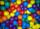You have 108 red and 180 green balls. You have to be grouped into the bags so that the ratio of red to green in each bag was the same. What smallest number of balls may be in one bag?
5. DivisorsThe sum of all divisors unknown odd number is 2112. Determine sum of all divisors of number which is twice of unknown numbers.
6. RemainderA is an arbitrary integer that gives remainder 1 in the division with 6. B is an arbitrary integer that gives remainder 2 the division by. What makes remainder in division by 3 product of numbers A x B ?
7. Sports games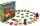Pupils of same school participated district sports games. When dividing into teams found that in the case of the creation teams with 4 pupils remaining 1 pupil, in the case of a five-member teams remaining 2 pupils and in the case of six-members teams r
8. Trees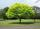Loggers wanted to seed more than 700 and less than 800 trees. If they seed in rows of 37, left them 8 trees. If they seed in rows of 43, left the 11 trees. How many trees must seed ?
9. Lines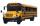Five bus lines runs at intervals 3, 6, 9, 12, 15 minutes. In the morning, suddenly start at 4:00. After how many minutes the bus lines meet again?
10. Sheep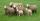Shepherd tending the sheep. Tourists asked him how much they have. The shepherd said, "there are fewer than 500. If I them lined up in 4-row 3 remain. If in 5-row 4 remain. If in 6-row 5 remain. But I can form 7-row." How many sheep have herdsman?
11. DivisibilityWrite all the integers x divisible by seven and eight at the same time for which the following applies: 100
12. Toy carsPavel has a collection of toy cars. He wanted to regroup them. But in the division of three, four, six, and eight, he was always one left. Only when he formed groups of seven, he divided everyone. How many toy cars have in the collection?
13. Shepherd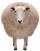The shepherd has fewer than 500 sheep; where they can be up to 2, 3, 4, 5, 6 row is always 1 remain, and as can be increased up to 7 rows of the sheep, and it is not increased no ovine. How many sheep has a shepherd?
14. The florist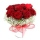The florist had 200 roses in the morning. During the day, more than half sold it. From the remaining roses, it will tie the bouquet. If a bouquet of 3, 4, 5 or 6 roses are bound, one always remains. How many roses from the morning shipment sold?
15. Ski tow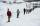The ski club has 168 pupils and used lift with 60 seats, while students always follow the same sequence in filling seats. How many times while riding a ski lift skier sitting in the same seat as the first run?
16. Children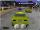Less than 20 children is played various games on the yard. They can create a pairs, triso and quartets. How many children were in the yard when Annie came to them?
17. GrandmotherGrandmother wants to give the candies to grandchildren that when she gives 5 candy everyone 3 missing and when she gives 4 candies 3 is surplus. How many grandchildren has grandmother and how many candies has?